Home    |    Teacher    |    Parents    |    Glossary    |    About UsIn this unit we'll be learning about equations in two variables. A coordinate plane is an important tool for working with these equations. It is formed by a horizontal number line, called the x-axis, and a vertical number line, called the y-axis. The two axes intersect at a point called the origin.

You can locate any point on the coordinate plane by an ordered pair of numbers (x,y), called the coordinates.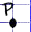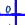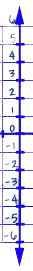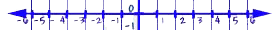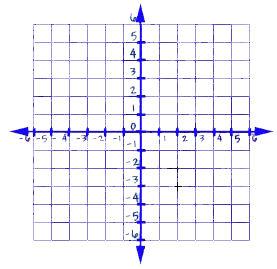Homework Help | Algebra | Graphing Equations and InequalitiesEmail this page to a friendSearch·  The coordinate plane·  Slope and y-intercept·  Graphing linear     equations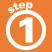First Glance In Depth Examples WorkoutThe coordinate plane